0%

## Graph-based SLAM

### 基本概念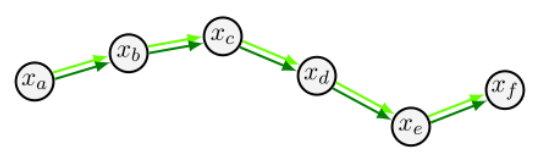### 图优化（后端）

• 通过相邻点之间的位置关系计算出 $T_{b,c,…,f}$
• 通过回环检测构建出来的边： $T_{bf}$

## 非线性最小二乘原理

### 问题引入

• $x$ 为该系统的状态向量，也是我们重点关注需要估计的值
• $z$ 为该系统的观测值，通常由传感器可以进行直接观测获得
• $f(x)$ 为该系统的关于状态向量 $x$ 的一组映射非线性函数，也称为观测函数，表示 $x$ 和 $z$ 之间的对应关系

### 求解方法

#### 总结

• 线性化误差函数： $e_i(\mathbf{x} + \Delta \mathbf{x}) = e_i(\mathbf{x}) + J_i\Delta \mathbf{x}$
• 构建线性系统：
• $b^T = \sum e_i^T\Sigma_iJ_i$
• $H = \sum J_i^T\Sigma_iJ_i$
• $H\Delta \mathbf{x} = b$
• 求解线性系统：$\Delta \mathbf{x}^* = -H^{-1}b$
• 更新解直至收敛：$\mathbf{x} = \mathbf{x} + \Delta \mathbf{x}^*$

## 非线性最小二乘在 SLAM 中的应用

### 图的构建

• 相邻节点之间的边通过里程计测量构建，这里的里程计可以是轮式里程计或者帧间匹配里程计（激光里程计），在视觉 SLAM 中也可以是视觉里程计；
• 不相邻节点之间的边要通过回环检测来构建，节点 i 和节点 j 观测到同样的环境信息,两者进行匹配得到相对位姿。并在对应的节点中连一条边,边为匹配的相对位姿。用信息矩阵来描述本次匹配的可靠性。

### 误差函数

• 预测观测值 $Z’_{ij}$：通过轮式里程计得到的相对位置关系可以作为相邻节点之间的预测值
• 实际观测值 $Z_{ij}$：通过两帧之间的帧间匹配得到的相对位姿可以作为实际观测值
• 因此误差函数 $e_{ij}(\mathbf{x}) = t2v(Z^{-1}Z’)$, $t2v$ 在这里表示位姿矩阵转换为位姿向量

### 线性化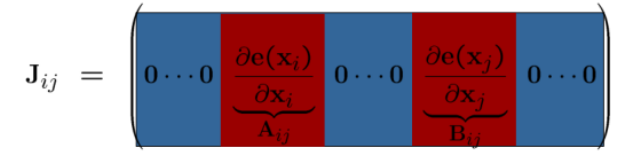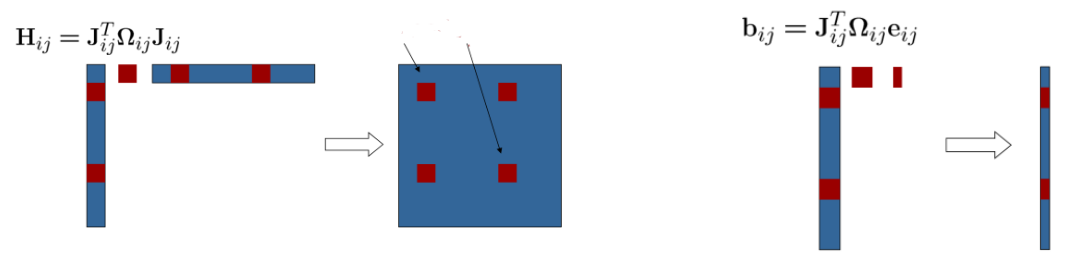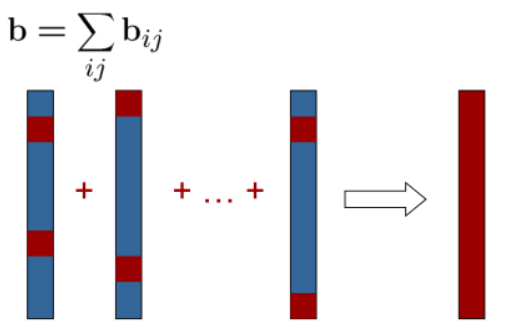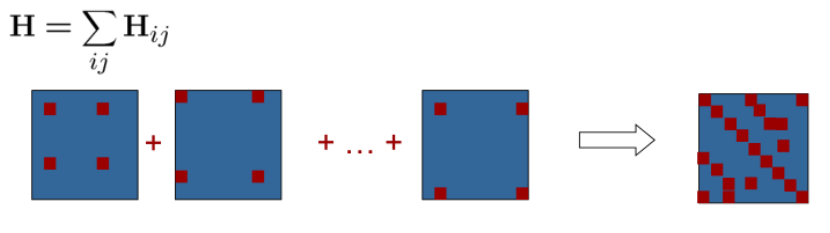### 构建线性系统

• 已知误差矩阵 $J$ 即 $(A{ij}, B{ij})$
• 向量 $b$ 的更新为：
• 矩阵 H 的更新为：

### 求解

• 已知矩阵 H 和 向量 b
• 求解 $H\Delta x = -b$，这里要利用稀疏矩阵的特殊性进行矩阵，不要直接求逆
• 进行迭代，直至收敛